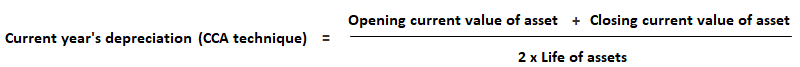In current cost accounting (CCA), assets are shown in the balance sheet at the current replacement costs after allowing for depreciation. This also requires an adjustment for depreciation.

### Formula

Use the following formula to calculate the current year’s depreciation under CCA:To calculate the depreciation adjustment, the following formula can be used:

Depreciation adjustment = Current year’s depreciation on CCA – Depreciation on historical cost

### Example

A machine was purchased on 1 January 2014 at a cost of \$1,000,000. Its useful life was estimated at 10 years. The machine’s replacement cost was \$180,000 on 1 January 2019 and \$2,000,000 on 31 December 2019. Calculate the depreciation adjustment.

#### Solution

Current year’s depreciation (CCA method) = (180,000 + 2,000,000) / (2 x 10)
= \$190,000
Historical depreciation = 1,000,000 / 10 = \$100,000
Depreciation adjustment = Current year’s depreciation on CCA – Historical depreciation
= 190,000 – 100,000
= \$90,0000

### What is the Depreciation adjustment if the book value of an asset is its original cost less accumulated Depreciation?

This is how it works: book value = (original cost – accumulated Depreciation) x (remaining useful life / estimated useful life) Depreciation adjustment = book value – historical book value = original cost – accumulation of Depreciation

### What is the Depreciation adjustment if the historical cost remains constant?

The Depreciation adjustment will be the historical cost - accumulated Depreciation.

### What is Depreciation?

Depreciation refers to the value lost by a certain good over a period of time due to wear and tear. It can also be called a diminution in value.

### When should Depreciation adjustment be used under CCA?

Depreciation adjustment is used while calculating Depreciation under the current Cost Accounting technique.

### What is CCA?

It stands for current Cost Accounting, which records assets at their replacement costs after allowing for Depreciation. Replacing assets would include buying new assets or refurbishing old ones.

True is a Certified Educator in Personal Finance (CEPF®), a member of the Society for Advancing Business Editing and Writing, contributes to his financial education site, Finance Strategists, and has spoken to various financial communities such as the CFA Institute, as well as university students like his Alma mater, Biola University, where he received a bachelor of science in business and data analytics.

To learn more about True, visit his personal website, view his author profile on Amazon, his interview on CBS, or check out his speaker profile on the CFA Institute website.